Environmental Corrections for Resistivity
Water saturation calculations require a good value for formation resistivity, commonly called true resistivity or Rt, as well as shale corrected porosity (PHIe), and the shale content (Vsh). Therefore, the resistivity log may need some environmental corrections before use in the saturation equations.

The corrections on this page are for older style induction logs and laterologs, and are shown for illustration purposes only. Use the appropriate charts for the specific tool that was run on your well. Note that invasion corrections, covered in the next Section, apply only to water zones and oil zone cases have not been published anywhere. On modern array induction logs, invasion corrections for both water and oil zone cases are applied by the service company to create Rt and Rxo curves. These algorithms have not been published.

Commercial log analysis software may use generic or obsolete environmental corrections, so care in the use of these corrections is required. No software package has individual corrections for every possible tool type from all service companies.

Borehole corrections for mud salinity and hole diameter should be applied first, if needed. Most computer aided log analysis software has this capability. Fortunately, borehole corrections can often be safely ignored when the log is run in a good borehole with a good mud system. The newest array induction logs attempt to produce Rt with all corrections applied. Do not over correct your data by applying the corrections a second time.Borehole Environment Corrections to Resistivity
For those who insist on superfluous detail, formulae are provided here for the deep induction log for 1.5 inches standoff, the usual case: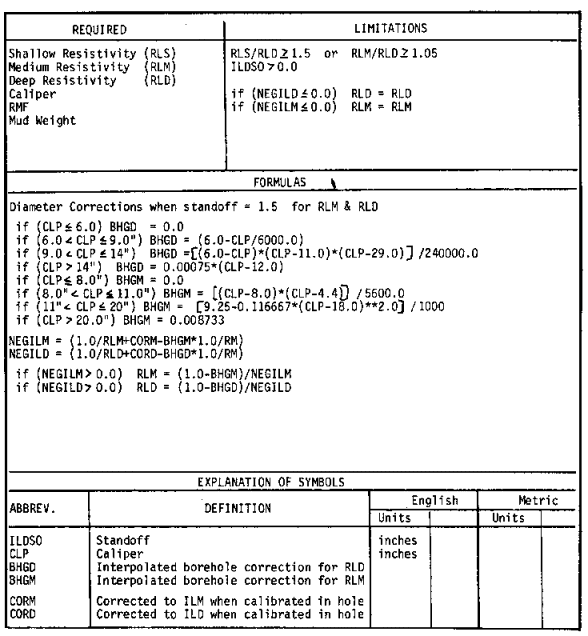Borehole correction for deep induction - standoff = 1.5 inches

Note that the abbreviations shown above are those used in this FORTRAN program and do not conform to abbreviations used in this Handbook. Hole size is in inches and correction to the code for metric dimensions is required. Charts for these formulae are given below for various tool types.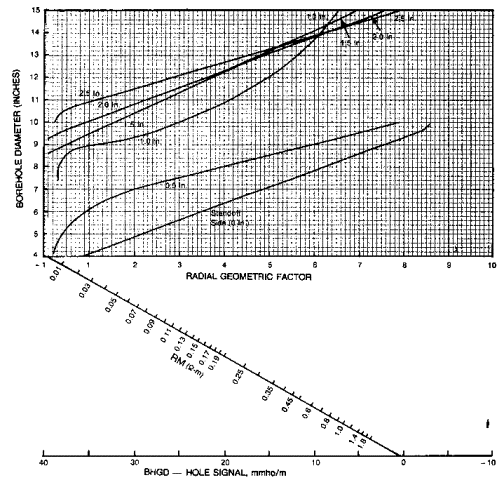Borehole correction for medium induction log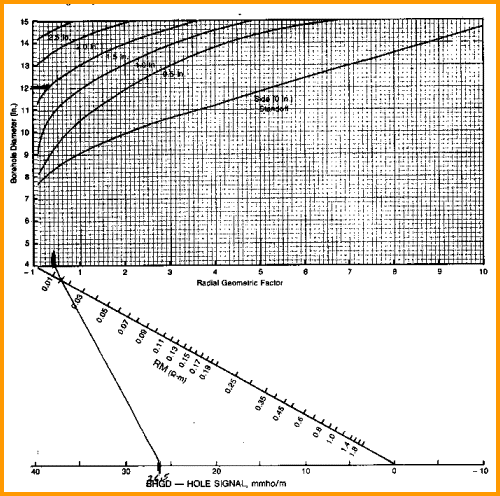Borehole correction for deep induction log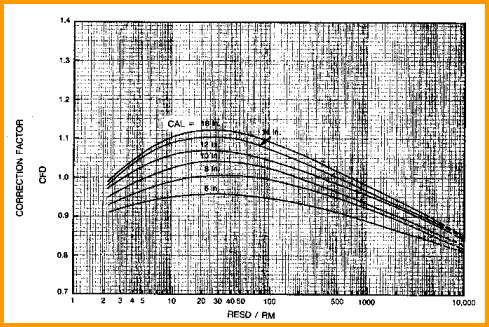Borehole correction for deep laterolog (dual)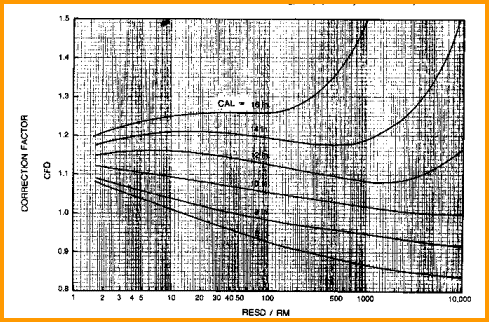Borehole correction for shallow laterolog (dual)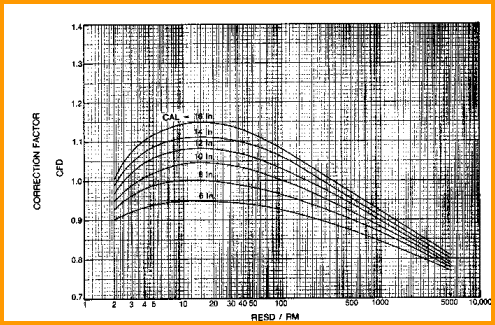Borehole correction for laterolog (single)

It is instructive to determine the borehole corrections for some typical cases and run a sensitivity study with one of the saturation equations to see if the corrections have a measurable impact.Induction Log Borehole Corrections
IES ang DIL ONLY

The borehole signal for induction logs is subtracted from the induction conductivity measurement and reciprocated to obtain corrected resistivity.
1: RESDc = 1000 / (1000 / RESD - BHGD)
2: RESMc = 1000 / (1000 / RESM - BHGM)

Where:
BHGD = deep resistivity correction (mS/m)
BHGM = medium resistivity correction (mS/m)
RESD = deep resistivity reading (ohm-m)
RESDc = deep resistivity corrected for borehole effect (ohm-m)
RESM = medium resistivity reading (ohm-m)
RESMc = medium resistivity corrected for borehole (ohm-m)

The values for BHGD and BHGM are to be taken from charts similar to those shown below, or from curve fits to these charts. Some computer programs use a look-up table. These charts are for Schlumberger’s 6FF40 tool. Different charts and look-up tables are needed for other induction logging tool designs.Laterolog Borehole Corrections
DLL Only
The borehole correction for laterologs is a correction factor which is divided into the original log reading to obtain the corrected value.
3: RESDc = RESD / CFD
4: RESMc = RESM / CFM
5: RESEDITFLAG\$ = "BH"

Where:
CFD = borehole effect correction factor for deep laterolog
CFM = borehole effect correction factor for shallow laterolog
RESD = deep resistivity reading (ohm-m)
RESDc = deep resistivity corrected for borehole effect (ohm-m)
RESM = medium resistivity reading (ohm-m)
RESMc = medium resistivity corrected for borehole (ohm-m)COMMENTS:
The values for CFD and CFM are to be taken from appropriate charts, or from curve fits to these charts. Some computer programs use a look-up table. These charts are for Schlumberger’s DLL tool. Different charts and look-up tables are needed for other laterolog tool designs.Invasion Corrections
The second correction is for the effects of invasion of mud filtrate into the formation. Knowledge of the invasion profile can be used to correct the deep resistivity log for this effect. The profile knowledge comes from the medium and shallow resistivity data when compared to the deep resistivity. Correction charts are available for various tool types, and are usually known as Tornado Charts due to the shape of the lines on the graph.

Sometimes the log data does not fit inside the body of lines on the chart. A pragmatic solution is to lower the deep resistivity by a an offset based on the difference between the logarithm of the shallow resistivity and the logarithm of the medium resistivity. An example is shown below. The equations to run standard invasion corrections follow. Note the warning at the end of this section.Original logs showing deep invasion on resistivity, with hydrocarbon volume (shaded red) and water saturation based on the deep resistivity as recorded.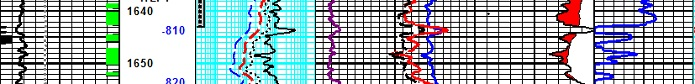Invasion corrected deep resistivity (dashed purple) is a linear shift of the logarithm of the original deep resistivity (dashed red). Note that the water zone now looks wet, the zone no longer triggers a pay flag, and original hydrocarbon -- water contact is now more clearly defined in this depleted reservoir.

In the above example, the need for invasion corrections is inferred from the fact that no oil or gas was produced from the perforations annotated at the top of the zone. If hydrocarbons had been produced, the deep resistivity would need to be increased. The published Tornado Charts for induction logs never increase resistivity, so they are meant only for wet or depleted intervals.Invasion Correction for Induction Logs
The invasion corrections for dual induction logs are computed as follows:
6: IF RESD < RESM
7: AND IF RESM < RESS
8: THEN H = RESS / RESD - 1
9: B = RESM / RESD - 1
10: C = H / B
11: D = 0.59 * H - 2.21 * C + 1.35
12: E = - 1.44 * H + 2.47 * C - 2.76
13: G = - 0.5 * ((D ^ 2 - 4 * E) ^ 0.5 + D)
14: IF RESD >= RESM
15: OR IF RESM >= RESS
16: THEN G = 1.0
17: RESDc = G * RESD

Where:
B = intermediate term
C = intermediate term
D = intermediate term
E = intermediate term
G = intermediate term
H = intermediate term
RESD = deep resistivity log reading (ohm-m)
RESDc = deep resistivity log reading corrected for invasion (ohm-m)
RESM = medium resistivity log reading (ohm-m)
RESS = shallow resistivity log reading (ohm-m)

If the medium and deep resistivity logs read the same value, then either no correction is needed because invasion is very shallow, or no correction is possible because invasion is extremely deep. These formulae are shown graphically below. Newer tools need different charts.

RESDc is often called Rt, the "true" resistivity - see warning below.NUMERICAL EXAMPLE:
1. For example, the data for Sand D gives:
RESS = 2.0
RESM = 1.5
RESD = 1.0
H = 2.0 / 1.0 - 1 = 1.0
B = 1.5 / 1.0 - 1 = 0.5
C = 1.0 / 0.5 = 2.0
D = 0.59 * 1.0 - 2.21 * 2.0 + 1.35 = -2.48
G = - 0.5 * ((2.48 ^ 2 - 4 * 0.74) ^ 0.5 - 2.48) = 0.35
RESDc = 0.35 * 1.0 = 0.35

Thus, invasion is so deep that the dual induction reads nearly three times too high. If this is a water zone, the correction is reasonable. If it is hydrocarbon bearing, the correction makes no sense.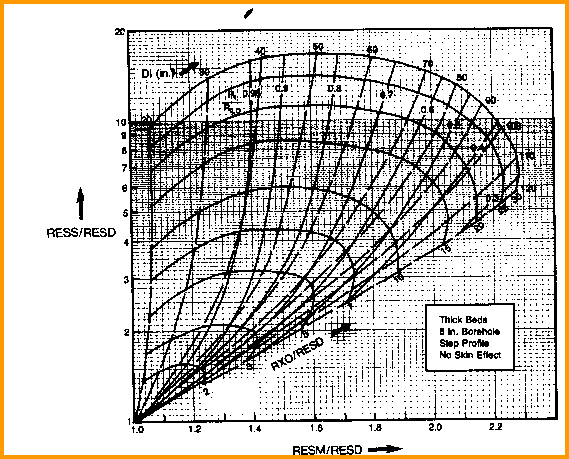Invasion correction for dual induction

 CRAIN'S OPINIONATED OPINION CAUTION: The invasion correction for induction logs as defined by service company charts always reduces Rt. This is fine in a water zone but is dead wrong in oil or gas zones, where Rt is usualy too low due to invasion of water. Lowering it even more is just too dumb for words. Therefore, DO NOT apply invasion corrections from these charts in hydrocarbon zones. Most software allows you to turn off these offensive corrections.

Below is a sample sensitivity analysis that shows the correction factor Rt/RESD is greater than 1.0 for many real situations. The same factor (Rt/Rild) on above graph is never greater than 1.0.

 SENSITIVITY ANALYSIS WATER SATURATION AND RESISTIVITY WITH INVASION
 Archie's Equation Sw = (A * RW@FT / (PHIe ^ M) / Rt) ^ (1 / N) Assume A=1.0, M = N = 2.0 Sw = (RW@FT / (PHIe ^ 2) / Rt) ^ 0.5 Rearrange terms Sw^2 = (RW@FT / (PHIe ^ 2) / Rt) Solve for Rt in uninvaded oil zone Rt= (RW@FT / (PHIe ^ 2) / Sw^2) Solve for Rxo in invaded oil zone Rxo= (RMF@FT / (PHIe ^ 2) / Sxo^2) Solve for R0 in uninvaded water zone R0= (RW@FT / (PHIe ^ 2) Assume RESD gets 50% of signal from invaded zone and 50% from uninvaded zone RESD = 1 / ((1 / Rt + 1 / Rxo) / 2) Solve for SWa in invaded oil or water zone Swa = (RW@FT / (PHIe ^ 2) / RESD) ^ 0.5 Multiply deep resistivity (RESD) by Rt/RESD ratio to obtain Rt from RESD

 INVADED OIL ZONE Sw=0.25 RMF@FT=1.000 Sxo=0.6 Sxo=0.8 Sxo=1.0 Sw=1.0 RW@FT PHIe Rt Rxo R0 RESD SWa Rt/RESD Rt/RESD Rt/RESD Rt/RESD 0.25 0.25 64.0 44.4 4.0 52.5 0.28 1.22 1.78 2.50 0.63 0.25 0.15 177.8 123.5 11.1 145.7 0.28 1.22 1.78 2.50 0.63 0.10 0.25 25.6 44.4 1.6 32.5 0.22 0.79 1.01 1.30 0.55 0.10 0.15 71.1 123.5 4.4 90.2 0.22 0.79 1.01 1.30 0.55 0.03 0.25 7.7 44.4 0.5 13.1 0.19 0.59 0.65 0.74 0.52 0.03 0.15 21.3 123.5 1.3 36.4 0.19 0.59 0.65 0.74 0.52

 INVADED OIL ZONE Sw=0.25 RMF@FT=0.50 Sxo=0.6 Sxo=0.8 Sxo=1.0 Sw=1.0 RW@FT PHIe Rt Rxo R0 RESD SWa Rt/RESD Rt/RESD Rt/RESD Rt/RESD 0.25 0.25 64.0 22.2 4.0 33.0 0.35 1.94 3.06 4.50 0.75 0.25 0.15 177.8 61.7 11.1 91.6 0.35 1.94 3.06 4.50 0.75 0.10 0.25 25.6 22.2 1.6 23.8 0.26 1.08 1.52 2.10 0.60 0.10 0.15 71.1 61.7 4.4 66.1 0.26 1.08 1.52 2.10 0.60 0.03 0.25 7.7 22.2 0.5 11.4 0.21 0.67 0.81 0.98 0.53 0.03 0.15 21.3 61.7 1.3 31.7 0.21 0.67 0.81 0.98 0.56

 INVADED OIL ZONE Sw=0.25 RMF@FT=0.25 RMF@FT Sxo=0.6 Sxo=0.8 Sxo=1.0 Sw=1.0 RW@FT PHIe Rt Rxo R0 RESD SWa Rt/RESD Rt/RESD Rt/RESD Rt/RESD 0.25 0.25 64.0 11.1 4.0 18.9 0.46 3.38 5.62 8.50 1.00 0.25 0.15 177.8 30.9 11.1 52.6 0.46 3.38 5.62 8.50 1.00 0.10 0.25 25.6 11.1 1.6 15.5 0.32 1.65 2.55 3.70 0.70 0.10 0.15 71.1 30.9 4.4 43.0 0.32 1.65 2.55 3.70 0.70 0.03 0.25 7.7 11.1 0.5 9.1 0.23 0.85 1.11 1.46 0.56 0.03 0.15 21.3 30.9 1.3 25.2 0.23 0.85 1.11 1.46 0.56

 INVADED OIL ZONE Sw=0.25 RMF@FT=0.10 RMF@FT Sxo=0.6 Sxo=0.8 Sxo=1.0 Sw=1.0 RW@FT PHIe Rt Rxo R0 RESD SWa Rt/RESD Rt/RESD Rt/RESD Rt/RESD 0.25 0.25 64.0 4.4 4.0 8.3 0.69 7.70 13.30 20.5 1.75 0.25 0.15 177.8 12.3 11.1 23.1 0.69 7.70 13.30 20.5 1.75 0.10 0.25 25.6 4.4 1.6 7.6 0.46 3.38 5.62 8.50 1.00 0.10 0.15 71.1 12.3 4.4 21.0 0.46 3.38 5.62 8.50 1.00 0.03 0.25 7.7 4.4 0.5 5.6 0.29 1.36 2.04 2.90 0.65 0.03 0.15 21.3 12.3 1.3 15.6 0.29 1.36 2.04 2.90 0.65Invasion Correction for Laterologs

The invasion corrections for dual laterologs are computed as follows:
18: IF RESD / RESS <= 1
19: THEN RESDc = 1.7 * RESD - 0.7 * RESM
20: IF RESD / RESM >= 1.1
21: THEN RESDc = 1.1 * RESD
22: C = RESM / RESS * (RESD - RESS) / (RESD - RESM)
23: IF C = 1 / 1.7
24: THEN RESDc = RESD
25: IF C # 1 / 1.78
26: THEN RESDc = 2.18 * C * RESD / (1.78 * C - 1)
27: OTHERWISE RESDc = RESD

Where:
C = intermediate term
RESD = deep resistivity log reading (ohm-m)
RESDc = deep resistivity log reading corrected for invasion (ohm-m)
RESM = medium resistivity log reading (ohm-m)
RESS = shallow resistivity log reading (ohm-m)

If the medium and deep resistivity logs read the same value, then either no correction is needed because invasion is very shallow, or no correction is possible because invasion is extremely deep. These formulae are shown graphically below. Newer tools need different charts.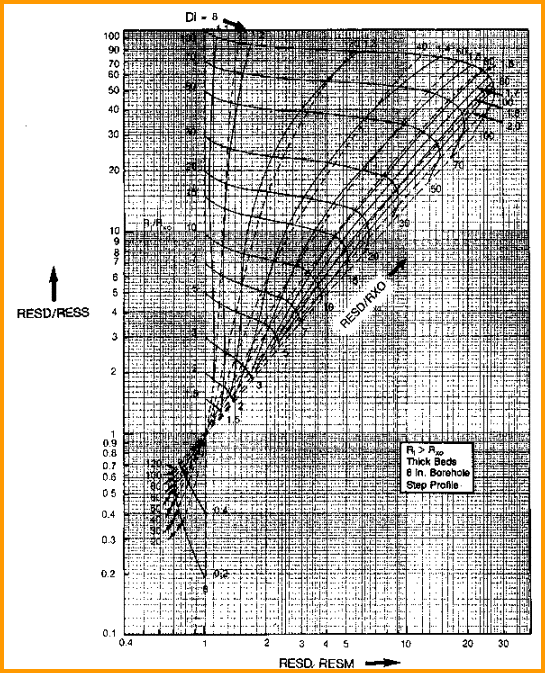Invasion correction for dual laterolog

This chart can raise or lower the Rt. Use the correction only if the correction raises Rt. The reader is encouraged to run a sensitivity analysis, similar to the one shown earlier for induction logs, for the laterolog in a salt mud case and a fresh mud case.NUMERICAL EXAMPLE:
1. Assume a dual laterolog had been run, the log might have read:
RESD = 2.0
RESM = 1.5
RESS = 1.0
C = 1.5 / 1.0 * (2.0 - 1.0) / (2.0 - 1.5) = 3.00
RESDc = 2.18 * 3.00 * 2.0 / (1.78 * 3.00 - 1) = 3.00Calculating Diameter of Invasion
The invasion correction described above can also be used to calculate an apparent invasion diameter (Di). On some modern logs, this result is calculated by the service company.  The generic formulae are:

For dual induction logs:
30: C = (RESM / RESDc) * (RESD - RESDc) / (RESM - RESD)
31: Di = 33 * (C + 1) - min (100, 10 ^ (0.5 * C - 0.04))

For dual laterolog:
32: IF RESDc / RESD > 1
33: THEN Di = 10 ^ (RESDc / RESD - 1)
34: IF RESDc / RESD < 1
35: THEN Di = 160 * (1 - RESD / RESDc)
36:: OTHERWISE Di = 0.0

Where:
Di = diameter of invasion (inches)
RESD = deep resistivity log reading (ohm-m)
RESDc = corrected deep resistivity reading (ohm-m)
RESM = medium resistivity log reading (ohm-m)

Di is in inches; multiply by 25.4 to get millimeters.

If RESDc / RESD = 1; Di cannot be determined.

While diameter of invasion is not used to correct other data, it is a useful quality control indicator.NUMERICAL EXAMPLE:
1. Data for Sand D gives:
RESS = 2.0
RESM = 1.5
RESD = 1.0
RESDc = 0.35 from previous example

C = (1.5 / 0.35) * (1.0 - 0.35) / (1.5 - 1.0) = 5.57
Di = 33 * (5.57 + 1) - min (100, 10 ^ (0.5 * 5.57 - 0.04)) = 116 inches

Page Views ---- Since 01 Jan 2015
Copyright 2023 by Accessible Petrophysics Ltd.
CPH Logo, "CPH", "CPH Gold Member", "CPH Platinum Member", "Crain's Rules", "Meta/Log", "Computer-Ready-Math", "Petro/Fusion Scripts" are Trademarks of the Author